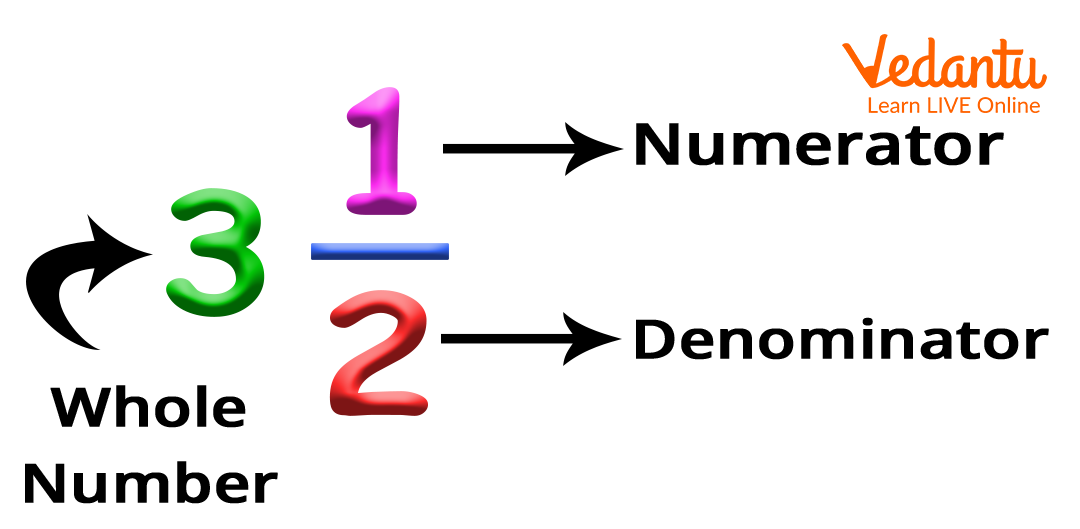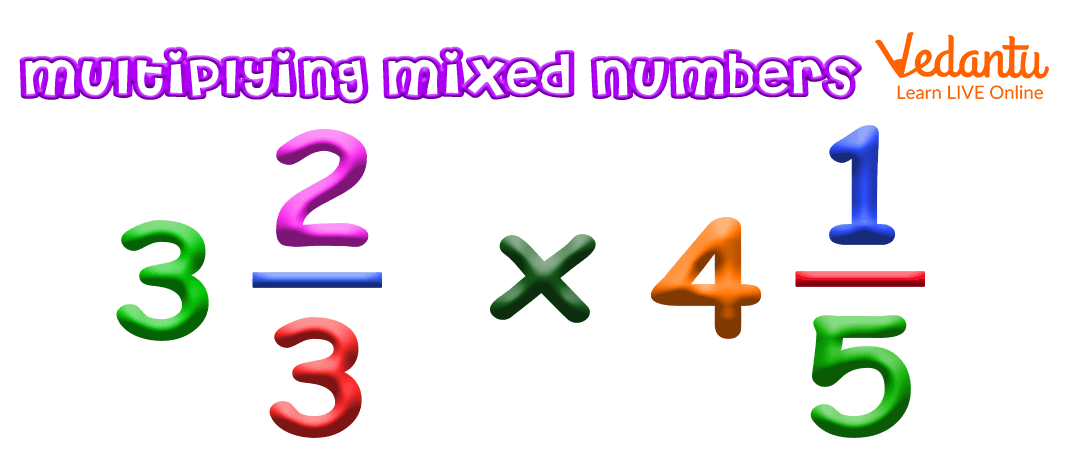Courses
Courses for Kids
Free study material
Free LIVE classes
More

# Multiplying Mixed NumbersLIVE
Join Vedantu’s FREE Mastercalss

## What Are Mixed Numbers?

Mixed numbers, also known as mixed fractions, are a combination of whole numbers and a proper fraction. The mixed number is partly a whole number and partly a fraction. The fractional part of a mixed number is always a proper fraction. For example, 534, 712, 723, etc are mixed numbers. Multiplying mixed numbers is similar to multiplying fractions. Here, we will learn the steps to multiply mixed numbers with the like denominators, unlike denominators, and whole numbers.

## Parts of Mixed Number

A mixed number is formed by combining three parts namely the whole number, a numerator, and a denominator.  Look at the image given to understand the parts of mixed numbers in a better way.## How to Multiply Two Mixed Numbers with Like and Unlike Denominators?

Two fractions with the same denominator are known as the like denominators. Contrarily, two fractions with different denominators are known as unlike denominators. The steps to multiply the mixed fractions remains the same irrespective of the like or unlike denominators. The 4 steps given below describes how to multiply mixed numbers with like and unlike denominators:

Step 1: Convert the given mixed numbers into improper fractions.

Step 2: Multiply first the numerators and and then the denominators of the improper fraction.

Step 3: Simplify the fraction or result obtained in the previous step in lowest terms if possible.

Step 4: Convert the result obtained back to the mixed number.## How to Convert a Mixed Number into an Improper Fraction?

To convert a mixed number into an improper fraction:

• Multiply the whole number with the denominator of the proper fraction.

• Add the numerator of the proper fraction to the product obtained in step 1.

• The denominator of the improper fraction will be the same as the denominator of the proper fraction.

• The resulting fraction will be an improper fraction.

Let us understand with an example:

Convert 512 into an improper fraction.

Solution:

Step 1: Multiplying the whole number with the denominator of the proper fraction.

5 2 = 10

Step 2: Adding the numerator of the proper fraction to the product obtained in step 1. Here, the numerator is 1. Accordingly, numerator of the  improper fraction will be  10 + 1 = 11

Step 3: The denominator of the improper fraction will stay the same. Accordingly, the denominator will be 2.

Hence, 512 in improper fraction will be  112.

As we have understood how to convert mixed number to an improper fraction, let us now understand multiplying mixed number with an example:

## Example on Multiplying Mixed Numbers with Like Denominators

What is 2 27 3 17 ?

Solution:

Step 1: Converting the mixed numbers into improper fractions, we have

### 3 17  = 3 7 + 17 = 227

Step 2: Multiplying the numerators and denominators of improper fractions obtained in step 1.

### 167227 = 35249

Step 3: Converting the final result back into the mixed fraction. Accordingly, 35249 = 7949

Therefore,  2 27 3 17 = 7949

## Example on Multiplying Mixed Numbers with Unlike Denominators

What is 1 23 2 34 ?

Solution:

Step 1: Converting the mixed numbers into improper fractions, we have

### 2 34  = 2 4 + 34 = 114

Step 2: Multiplying the numerators and denominators of improper fractions obtained in step 1.

### 53114 = 5512

Step 3: Converting the final result back into the mixed fraction. Accordingly, 5512 = 4712

Therefore, 1 23 2 33  = 4712

## How to Multiply Mixed Numbers with the Whole Number?

The steps on multiplying the mixed number with the whole number are discussed below:

Step 1: Convert the whole number into fraction assuming the denominator to be 1.

Step 2: Convert the given mixed numbers into improper fractions.

Step 3: Multiply the numerators and denominators of the fraction.

Step 4: Simplify the result or fraction obtained in the previous step in lowest terms if possible.

Step 5: Convert the result obtained back to the mixed number.

Let us understand with an example:

What is 216 3?

Solution:

Step 1: Converting the whole number into fraction assuming the denominator to be 1. Hence, 3 will be 31

Step 2:  Converting the given mixed number into improper fraction gives:   216 = 136

Step 3: Multiplying the numerators and denominators of the fraction gives:

136 31 = 396

Step 4: Simplifying  the fraction obtained in the previous step in lowest terms gives:

396 = 39 36 3  = 132

Step 5: Converting the result obtained back to the mixed number gives:

132 = 6 12

Therefore, 216 3 = 6 12

In short, multiplying mixed numbers with fractions is a four-step process. Here, first you have to convert a given mixed number into an improper fraction, multiply the numerator and denominator of the fraction. Simplify the result if possible and convert the result back to the mixed number.

Last updated date: 23rd Sep 2023
Total views: 139.5k
Views today: 1.39k

## FAQs on Multiplying Mixed Numbers

1. How to convert improper fraction into mixed numbers?

To convert improper fraction into mixed number, divide the numerator by denominator. Now, consider the quotient as the whole number, and the remainder as the numerator of the proper fraction keeping the denominator same.  For example,  in 192, divide 19 by 2 to get quotient value as 9 and remainder value as 1. Accordingly, 192 = 9 12.

2. What is known as simplifying fraction in lowest possible terms in multiplying mixed numbers?

Simplifying fractions in lowest terms involves dividing both the numerator and denominator by a common factor to minimise the fraction to their equivalent fraction with the smallest possible numerator and denominator.